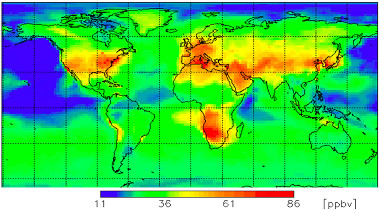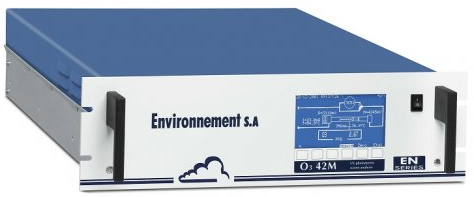﻿Ozone AQI: Using concentrations in milligrams or ppb?
 Trang Chủ Here bản đồ mặt nạ Câu hỏi thường gặp tìm kiếm Liên hệ Liên kết

Ozone AQI: Using concentrations in milligrams or ppb?

Posted on September 6th 2015
Share: aqicn.org/faq/2015-09-06/ozone-aqi-using-concentrations-in-milligrams-or-ppb

The ambient Ozone, refered as O3 and also called called ground-level or tropospheric Ozone impacts everyone on earth regardless of the country, as shown on the image on the right .

Afternoon ground level Ozone concentration in July 2011(Attribution: WMO GAW research on reactive gases )

Unlike particulate matter (PM2.5), the ground-level Ozone is not emitted directly. It is instead produced through a series of chemical reactions that occur in presence of nitrogen oxides, volatile organic compounds, sunlight and high temperatures, as shown on the following visual:Quantifying the impact of this ground level Ozone on Health is done via the Air Quality Index standard which each countries defines. What is interesting is that half of the world is using a standard based on milligrams measurement, while the rest is using ppb based measurement. But is this really a problem? This is what we will be looking at in this article.

--

The US EPA standard for Ozone is based on ppm, while the European one are based on milligrams.So, we naturally targetted our question to Environnement S.A, one of the leading European enviromental equipment manufacturer, who develops its own O342M Ozone analyser (see picture on the right). The O342M is both both certified by the US EPA and the EU (see specsheet), therefore able to provide measurement in both ppm and milligrams. Our question to Environnement S.A was 'How does your ozone analyser support both ppm and milligrams output? Is there any hardware difference for measurement? If not, is there any standard for the convertion?'.

Ozone Measurement PrinciplesThe ozone measurement is based on the universally known UV absorption principle , which consists in measuring UV absorption of ozone molecules. Ozone concentration is determined by difference between UV absorption of the gas sample and the sample without ozone after filtration performed by a catalytic converter.

In this system, the Ozone concentration is measured as an amount of light energy per volume of air, from which the concentration in ppbv is deducted. The lower detectable limit for this system is 0.4 ppb (corresponding to an AQI of 0.3, based on the US EPA 8-hour Ozone standard). This system does not measure the mass as such, but there is a standard way of doing the convertion from ppmv to mg/m3.

Converting Atmospheric Pollutant Concentrations: from ppmv to mg/m3

First, ppm (parts per million  ) and ppb (parts per billion) are defined as 1 ppm = 1/106 = 10-6 and 1 ppb = 1/109 = 10-9. So 1 ppm = 1000 ppb or 1 ppb = 10-3 ppm.

The conversion factor depends on the temperature at which you want the conversion (usually 25 degrees Centigrade in the US), as well as the ambient pressure. At an ambient pressure of 1 atmosphere, the general equation is:

$$c = { ppmv \times 12.187 \times MW \over 273.15 + t }$$
where:
• c = concentration in mg/m3(i.e., milligrams of gaseous pollutant per cubic meter of ambient air)
• MW = molecular weight of the gaseous pollutant
• ppmv = parts per million by volume (i.e., volume of gaseous pollutant per million volumes of ambient air)
• t = ambient temperature in degrees centigrade.
• 12.187 = inverse of the Universal Gas Law constant
As an example, for gaseous pollutant O3 (ozone), to convert 20 ppmv of Ozone to mg/m3 at 25 °C and 1 atmophere, the following formula is used:

$$c(20 ppmv) = { 20 \times 12.187 \times 48 \over 273.15 + 25 } = {20 \times 1.97} = 39.4 mg/m^3$$

where: 48.00 = MW(O3) = molecular weight of Ozone O3

European and US Convertion Standards

The assumption on the ambient temperature and atmospheric pressure is actually standardized and summurized in the following table for US, Europe or normal conditions. For our O342M Ozone analyzers, this coefficient is user programmable by the operator of the instrument.

 Gas Standard Conditions for Temperature and Pressure ( STP) "STP US" Conditions at 25°C (US EPA standard)  1013 mbar and 298K "STP European Union" Conditions at 20°C (EU standard)  1013 mbar and 293K "Normal" Conditions at 0°C 1013 mbar and 273K O3 - Ozone 1 ppb = 1,97 µg/m3 1 ppb = 2,00 µg/m3 1 ppb = 2,15 µg/m3 NO2 - Nitrogen Dioxyde 1 ppb = 1,88 µg/m3 1 ppb = 1,91 µg/m3 1 ppb = 2,05 µg/m3

Note: For those interested in knowing why 20°C is used as the standard reference temperature, you can check Ted Doiron's article on "20 °C—A short history of the standard reference temperature for industrial dimensional measurements".
Many thanks to Serge from Environnement S.A for the quick and precise answer on the Ozone monitors. Note that the same concept also applies to other gases such as Nitrogen Dioxide (for instance using the AS32M analyser).

--

To push the investigation a bit further, the next question is what would be the impact on the calculated Air Quality Index if the actual temperature and pressure would be used instead of the reference one?

Impact from ambient temperature

The last question is to look at the impact of a temperature variation on the Air Quality Index. For instance, let assume that an instrument is measuring an average of 120 mg/m3 of Ozone over 1 hour, which corresponds to an AQI of 50 (Medium) according to the European Common Air Quality Index (CAQI).

At 20°C and 1 atm, 120 mg/m^3 converts to 120/2.00, i.e. 60.0 ppmv. So let us assume that this is the actual measurement from the Ozone sensor. The question is then, what if the ambient temperature was peaking to 42°C, as it sometimes happen during summer heat waves, then what would be the correct mass? The convertion formula is:

$$c = { ppmv \times 12.187 \times MW \over 273.15 + t } = 111.37$$
This results in a difference of 8.6 mg/m^3 of measured Ozone. When applying the CAQI standard, the corresponding AQI is 46.4 (instead of 50 using the standard 20°C condition). This is actually an acceptable difference.

The generalized adjustement formula depending on the ambient temperature is summurized with the graph on the right. The x-axis is the ambient temperature, and the y-axis the calculated AQI is the actual temperature would be used instead of the reference one (20°C).

Impact from atmospheric pressure

As concerns the atmospheric pressure, the varation is defined by the Ideal Gas Law (PV = nRT). The value 12.187 is actually the inverse of the Universal Gas Law constant R. So, to understand the impact of atmospheric pressure, the following formula can be used:

$$R = {{ P \times V } \over {n \times T}} = {{ P \times 22.4 } \over { 1 \times 273 }} = { P \over 12.1875 }$$
In other words, one just need to divide the convertion factor by the current atmosphere. Assuming that the pressure p is expressed in millibars (1 atm = 1013.25 mb), the generalized convertion formula becomes:

$$c = { ppmv \times MW \times 1013.25 \times 12.1875 \over { p \times (273.15 + t)} }$$

where:
• c = concentration in mg/m3(i.e., milligrams of gaseous pollutant per cubic meter of ambient air)
• MW = molecular weight of the gaseous pollutant
• ppmv = parts per million by volume (i.e., volume of gaseous pollutant per million volumes of ambient air)
• t = ambient temperature in degrees centigrade.
• p = ambient atmospheric pressure in millibars.

Conclusions

The above explanations confirm our initial hypothesis that even though Ozone readings can be provided with different units (ppm and mg/m^3), this is actually not a problem since there is a standard way to convert readings from mg/m^3 to ppm and vice-versa. Moreover, the impact of using the reference STP (Standard Temperature & Pressure) instead of the actual ambient temperature and pressure is minimal, i.e. just units of Index in difference for the Ozone.

Credits: Ozone visual recreated using Icon pack by Icons8 and taken from American Chemical Society.

 This Ozone is not to be confused with the good ozone also called stratospheric ozone
 Parts per million also can be expressed as milligrams per liter (mg/L). This measurement is the mass of a chemical or contaminate per unit volume of water. Seeing ppm or mg/L on a lab report means the same thing. from University of Minnesota
 https://en.wikipedia.org/wiki/Gas_constant

• Nitrogen Dioxyde (NO2) in our atmosphere
• Ozone AQI Scale update
• Kriging Interpolation

Về phương pháp đo chất lượng không khí và mức độ ô nhiễm:

Về các thang đo chất lượng không khí

 - Giá trị chỉ số chất lượng không khí (AQI) Mức độ ảnh hưởng sức khỏe 0 - 50 Tốt Chất lượng không khí được xem là đạt tiêu chuẩn, và ô nhiễm không khí coi như không hoặc gây rất ít nguy hiểm 51 -100 Trung bình Chất lượng không khí ở mức chấp nhận được; tuy nhiên, một số chất gây ô nhiễm có thể ảnh hưởng tới sức khỏe của một số ít những người nhạy cảm với không khí bị ô nhiễm. 101-150 Không tốt cho người nhạy cảm Nhóm người nhạy cảm có thể chịu ảnh hưởng sức khỏe. Số đông không có nguy cơ bị tác động. 151-200 Có hại cho sức khỏe Mỗi người đều có thể sẽ chịu tác động đến sức khỏe; nhóm người nhạy cảm có thể bị ảnh hưởng nghiêm trọng hơn. 201-300 Rất có hại cho sức khỏe Cảnh báo nguy hại sức khỏe nghiêm trọng. Đa số mọi người đều bị ảnh hưởng. 300+ Nguy hiểm Cảnh báo sức khỏe: tất cả mọi người có thể chịu tác động nghiêm trọng đến sức khỏe

Để biết thêm về ô nhiễm và chất lượng không khí , xem wikipedia chất lượng không khí đang đề hoặc hướng dẫn về chất lượng không khí và sức khỏe của bạn .

Để biết những lời khuyên về sức khỏe rất hữu ích của bác sĩ y khoa Richard Saint Cyr ở Bắc Kinh, xem www.myhealthbeijing.com blog.

Thông báo sử dụng: Tất cả dữ liệu chất lượng không khí không được xác thực tại thời điểm công bố. Nhằm đảm bảo chất lượng, những dữ liệu này có thể được cập nhập mà không cần thông báo trước. Nhóm dự án Chỉ số chất lượng không khí toàn cầu đã thực hiện tất cả yêu cầu cần thiết trong việc biên soạn các thông tin này. Nhóm dự án hoặc các bên liên quan sẽ không chịu trách nhiệm về bất kỳ tổn thất, thương tích hoặc thiệt hại nào phát sinh trực tiếp hoặc gián tiếp từ việc cung cấp những dữ liệu này trong bất kỳ trường hợp nào.

Cài đặt

Cài đặt ngôn ngữ:

 Temperature unit:Celcius